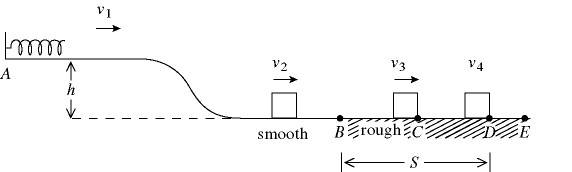# Energy Conservation & Potential Energy Spring/Friction Problem

## Homework Statement

A 0.80-kg block is held in place against the spring by a 67-N horizontal external force. The external force is removed, and the block is projected with a velocity v1 = 1.2 m/s upon separation from the spring. The block descends a ramp and has a velocity v2 = 1.9 m/s at the bottom. The track is frictionless between points A and B. The block enters a rough section at B, extending to E. The coefficient of kinetic friction is 0.39. The velocity of the block is v3 = 1.4 m/s at C. The block moves on to D, where it stops. In Fig. 7.4, the force constant of the spring is..## Homework Equations

K1 +U1 +W other = K2+ U2.. ect .. U2=0.5kx^2 .. K1=(0.5)mv^2

## The Attempt at a Solution

I've tries solving the problem with the equation above but I really just don't know where to start especially since there are no units of distance given.. Can someone lead me in the right direction please?

Last edited:

## Answers and Replies

tiny-tim
Science Advisor
Homework Helper
Hi Help1212!(try using the X2 and X2 icons just above the Reply box)

I assume you can find kx and kx2/2 ?

ok, then k = … ?euhh... i'm still a little confused..how do i find k if I don't know x?

tiny-tim
Science Advisor
Homework Helper
x is twice kx2/2 divided by kxhmm. well when I move around the equation i get k=2[0.5(mv22)/x2

from the assumption that K1 + U1 = K2 + U2

Am i going in the right direction??

tiny-tim
Science Advisor
Homework Helper
can you find kx and kx2/2 ?

if so, what are they?